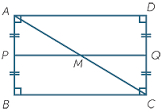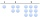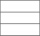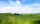# Rectangle area

What is the area of a rectangle that has a length of 4 feet and a width of 4/10 feet?

A =  1.6 ft2

### Step-by-step explanation:Did you find an error or inaccuracy? Feel free to write us. Thank you!#### You need to know the following knowledge to solve this word math problem:

We encourage you to watch this tutorial video on this math problem:

## Related math problems and questions:

• An architectAn architect makes a model of a new house. The model shows a tile patio in the backyard. In the model, each tile has a length of 1/2 inch and a width of 1/6 inch. The actual tiles have a length of 2/3 feet and a width of 2/9 feet. What is the ratio of the
• Father's gardenJohn's father has a garden in the back yard. The dimensions of the garden are shown here. A green rectangle is shown. The width is labeled as 2 and two-thirds feet. The length is labeled as 7 and three-fourths feet. Calculate the area of the garden. You f
• AlexandraAlexandra made a rectangular quilt the measured 3 1/4. 2 3/4 feet in width. What is the area of the quilt in square feet? Write an equation to solve.
• Find areaFind area of a rectangle with a length of 58 foot and a width of 49 foot?
• Area of rectangleHow many times will increase the area of the rectangle if we increase twice the length and at the same time we decrease the width by half?
• Length 7Length equals 7/6 inch in width equals 7/9 inches the area is?
• The length 2The length of a rectangle is 12y2 – 15y + 8 and the width is 7y – 11. Find the area of the rectangle
• SandboxSandbox has area of 32 sq ft and length of 4 1/2 ft. What is width of sandbox.
• Jared's room paintingJared wants to paint his room. The room's dimensions are 12 feet by 15 feet, and the walls are 9 feet high. Two windows measure 6 feet by 5 feet each. There are two doors, whose dimensions are 30 inches by 6 feet each. If a gallon of paint covers approxim
• LandLand has a rectangular shape, its surface area is 1.45 hectares. Its width is 250 m. Determine the length of the land.
• AmeekaAmeeka is in charge of designing a school pennant for spirit week. She wants the base to be 3 1/2 feet and the height to be 6 1/2 feet. She has 20 square feet of paper available. Does she have enough paper?
• A rectangleA rectangle has an area of 36 cm2. What could the length and width of rectangle be?
• Rectangle 45The perimeter of a rectangle is 60cm. If the length of the rectangle is 20cm. a)find the width b)find the area.
• A rectangle'sA rectangle's length is 3 times more than twice its width. If the perimeter of the rectangle is 60 feet. What is the length of the rectangle
• GardenThe rectangular garden has a length 99 m and width 84 m. Calculate how many m2 will decrease its area if land by the ornamental fence with width 30 cm.
• BackyardCorey's backyard is rectangular with a length of 23 miles. If the area of the backyard is 12 square miles, what is its width?
• RectangleCalculate area of the rectangle if its length is 12 cm longer than its width and length is equal to the square of its width.